# Addition of Three 2-digit numbers with Regrouping | Maths Grade 2

### Addition of Three 2-digit numbers with Regrouping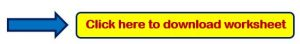Example 1:- Add the following?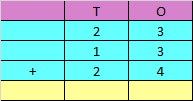Explanation:

Step 1: Adding the digits at one’s place,

3 + 3 + 4 = 10

Since the number is more than 9, we need to carry over 1 to the tens place.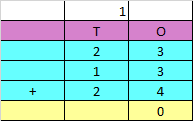Step 2: Adding the digits at tens place and the carry of 1,

2 + 1 + 2 + 1 = 6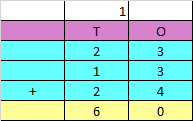Hence, the sum of the given numbers is 60

Example 2:- Add the following?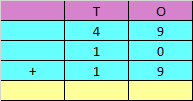Step 1: Adding the digits at one’s place,

9 + 0 + 9 = 18

Since the number is more than 9, we need to carry over 1 to the tens place.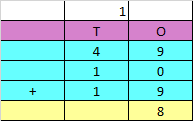Step 2: Adding the digits at tens place and the carry of 1,

4 + 1 + 1 + 1 = 7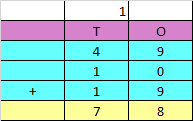Hence, the sum of the given numbers is 78

Example 3:- Add the following?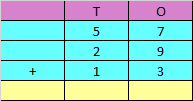Explanation:

Step 1: Adding the digits at one’s place,

7 + 9 + 3 = 19

Since the number is more than 9, we need to carry over 1 to the tens place.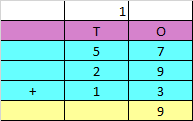Step 2: Adding the digits at tens place and the carry of 1,

5 + 2 + 1 + 1 = 9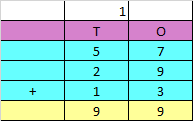Hence, the sum of the given numbers is 99